# Solution assignment 04 Fractional functions and graphs

### Assignment 4

Given the function: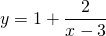Find:
the vertical asymptote;
the horizontal asymptote;
the intersection point with the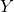-as if it exists;
the intersection point with the-as if it exists.

Based on these results sketch the result in the figure.

### Solution

The vertical asymptote of this function is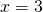. We find the horizontal asymptote by takingor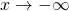. We get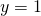because the second term approaches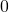and then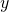approaches. The intersection point with the-axis is found by substituting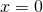in the formula. We get the intersection point with the-axis by substituting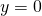and solving the equation: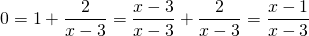The solution is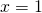0